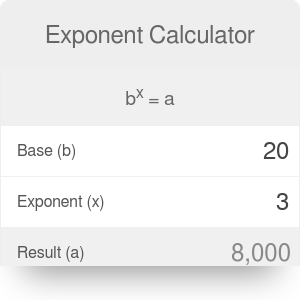The base (b)
to the power (x) of
equals (a)

# Exponent Calculator

By Mateusz Mucha and Piotr Małek

The exponent calculator will calculate the value of any base raised to any power. This page will cover all the related topics, including the negative exponent. Let's start with the basics.

## What is an exponent?

An exponent is a way to represent how many times a number, known as the base, is multiplied by itself. It is represented as a small number in the upper right hand corner of the base. For example: `x²` means you multiply x by itself two times, which is `x * x`. Likewise, `4² = 4 * 4`, etc. If the exponent is 3, in the example `5³`, then the result is `5 * 5 * 5`.

It's easy with small numbers, but for bases that are large numbers, decimals, or when they are raised to a power that's very large or negative, use our tool. If you wish to do exponentiation by hand, do the following:

1. Determine the base and the power it's raised to, for example `3⁵`.
2. Write the base the same number of times as the exponent. `3 3 3 3 3`
3. Place a multiplication symbol between each base. `3 * 3 * 3 * 3 * 3`.
4. Multiply! `3 * 3 * 3 * 3 * 3 = 243`.

## Negative exponent calculator

The concept is rather simple when the exponent is positive, but what happens when the exponent is negative? By the definition, if it is -2, we would multiply the base times itself negative two times. In actuality, what is happening here, we take the reciprocal of the base and change the negative exponent to positive and proceed as usual. If you'd like to work it out by hand, do the following:

1. Determine the base and the exponent.
2. Write the reciprocal of the base and change the sign of the exponent to positive
3. Write the reciprocal of the base the same number of times as the exponent.
4. Place a multiplication symbol between each.
5. Multiply and get the result.

Here's a quick example: `5⁻⁴ = (1/5)⁴ = (1/5) * (1/5) * (1/5) * (1/5) = 1/625 = 0.0016`

Squaring a base (raising a number to the power of 2) and taking the square root are similar concepts, many people consider one the opposite or the undoing of the other. If you want to square the number 6, you take `6 * 6 = 36`. Now if you want to find what two identical numbers multiply to give you 36, you take the square root of 36. This square root gives the value of 6. It can also be noted that squaring a square root removes the radical.

In case you need to calculate the cube root you can use our cube root calculator which is an excellent tool that will calculate the cube root of any number.

Besides, you may check our logarithm calculator which is the inverse function of the exponent.

Mateusz Mucha and Piotr Małek

## Get the widget!

Exponent Calculator can be embedded on your website to enrich the content you wrote and make it easier for your visitors to understand your message.

It is free, awesome and will keep people coming back!